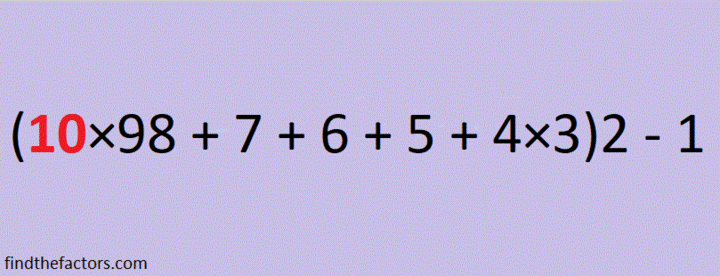# Facts about and Factors of 2019

Here’s a countdown you can use to ring in the New Year:make science GIFs like this at MakeaGif

2019 is the sum of consecutive numbers three different ways:
1009 + 1010 = 2019
672 + 673 + 674 = 2019
334 + 335 + 336 + 337 + 338 + 339 = 2019

There is one way that 2019 is the sum of consecutive odd numbers:
671 + 673 + 675 = 2019

2019 is the difference of two squares two different ways:
338² – 335² = 2019
1010² – 1009² = 2019

2019 is the sum of three squares nine different ways:
43² + 13² + 1² = 2019
43² + 11² + 7² = 2019
41² + 17² + 7² = 2019
41² + 13² + 13² = 2019
37² + 25² + 5² = 2019
37² + 23² + 11² = 2019
37² + 19² + 17² = 2019
35² + 25² + 13² = 2019
31² + 23² + 23² = 2019

2019 is the hypotenuse of a Pythagorean triple:
1155-1656-2019 so 1155² + 1656² = 2019²

2¹⁰ + 2⁹ + 2⁸ + 2⁷ + 2⁶ + 2⁵ + 2¹ + 2⁰ = 2019

2019 is a palindrome in a couple of bases:
It’s 5B5 in BASE 19 (B is 11 base 10) because 5(19²) + 11(19) + 5(1) = 2019,
and 3C3 in BASE 24 (C is 12 base 10) because 3(24²) + 12(24) + 3(1) = 2019

Every year has factors that often catch people by surprise. Today I would like to give you my predictions for the factors of 2019:
2019 will have four positive factors: 1, 3, 673, and 2019
However, 2019 will also have four negative factors: -1, -3, -673, and -2019

Which factors, positive or negative, will be your focus in the coming year?Finally, I’ll share some mathematics-related 2019 and New Year tweets that I’ve seen on twitter. Some of these tweets have links that contain even more facts about the number 2019.

Advertisements

## 2 thoughts on “Facts about and Factors of 2019”

1.Edmark M. Law

2019 = (1 + 2) × 3 + (45 + 67 + 89) × 10
= 10 × (−9 + (8 + 7) × (−6 + 5 × 4)) + 3 × (2 + 1)
= 0³ + 1⁸ + 2⁷ – 3⁹ + 4⁶ + 5⁴ + 6² + 7⁵ + 8¹ + 9⁰ (pandigital)
= 16 + 442 + 750 + 811 = 1⁶ + 44² + 75⁰ + 81¹

•ivasallay

Thank you for these and all the other ones you put on your website!

This site uses Akismet to reduce spam. Learn how your comment data is processed.